Equations and Lengths of Tangents and NormalsCustom SearchEQUATIONS AND LENGTHS OF TANGENTS AND NORMALS In figure 3-5, the coordinates of point P1 on the curve are (x1,y1). Let the slope of the tangent line to the curve at point P1 be denoted by m1. If you know the slope and a point through which the tangent line passes, you can determine the equation of that tangent line by using the pointŁslope form. Thus, the equation of the tangent line, MP1, is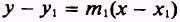The normal to a curve at a point (x1,y1) is the line perpendicular to the tangent line at that point. The slope of the normal line, m2, is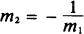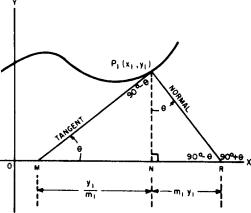Figure 3-S.-Curve with tangent and normal lines. where, as before, the slope of the tangent line is m1. This is shown in the following: If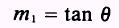thentherefore,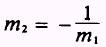The equation of the normal line through P1 is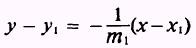Notice that since the slope of the tangent line is m, and the slope of the line which is normal to the tangent is m2 andthen the product of the slopes of the tangent and normal lines equals -1. The relationship between the slopes of the tangent and normal lines is stated more formally as follows: The slope of the normal line is the negative reciprocal of the slope of the tangent line. Another approach to show the relationship between the slopes of the tangent and normal lines follows: The inclination of one line must be 900 greater than the other. Then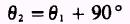ifthenWe know from trigonometry that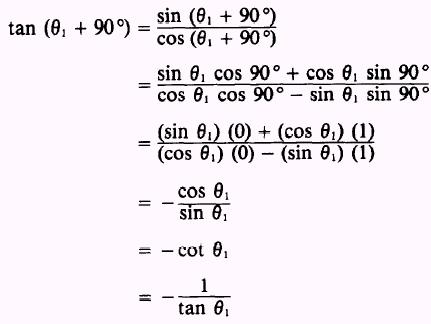Therefore,The length of the tangent is defined as that portion of the tangent line between the point P1 (x1,y1) and the point where the tangent line crosses the X axis. In figure 3-5, the length of the tangent is the line MP,. The length of the normal is defined as that portion of the norŁmal line between the point P1 and the X axis; that is the line, P1R1 which is perpendicular to the tangent line. As shown in figure 3-5,.the lengths of the tangent and normal may be found by using the Pythagorean theorem. From triangle MP1N, in figure 3-5,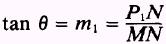andSincethen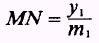In triangle NP1R,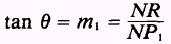andThus, the length of the tangent is equal to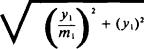and the length of the normal is equal toEXAMPLE. Find the equation of the tangent line, the equaŁtion of the normal line, and the lengths of the tangent and the normal of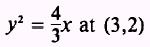SOLUTION: Find the value of 2a fromSince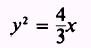then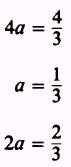The slope is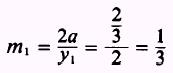Using the point-slope form of a straight line, we have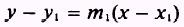then, at point (3,2)and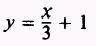which is the equation of the tangent line. Use the negative reciprocal of the slope to find the equation of the normal line as follows:thenTo find the length of the tangent, we use the Pythagorean theorem. Thus, the length of the tangent is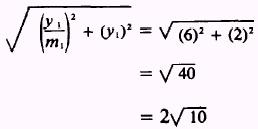The length of the normal is equal to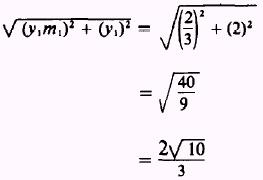PRACTICE PROBLEMS: Find the equations of the tangent line and the normal line, and the lengths of the tangent and the normal for the following: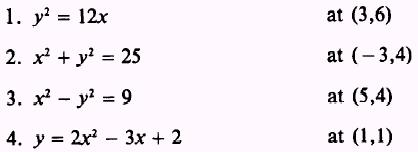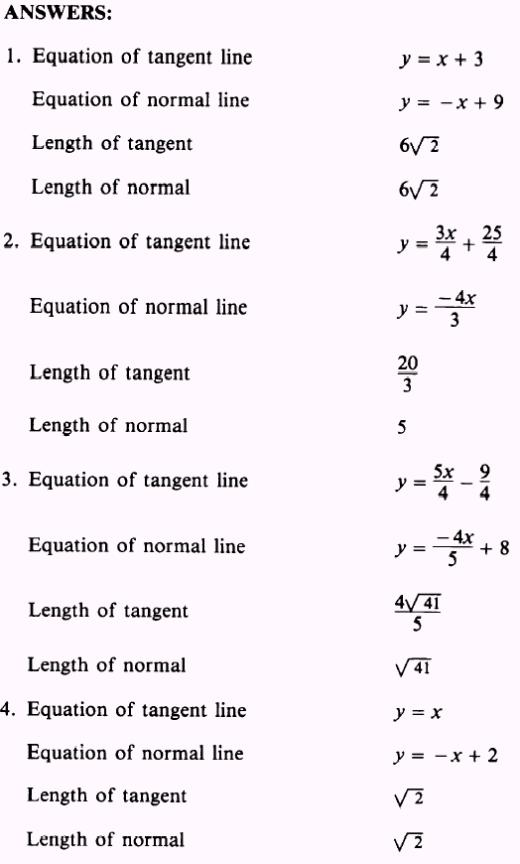Integrated Publishing, Inc. - A (SDVOSB) Service Disabled Veteran Owned Small Business